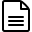# How is the score in the Listings section of Report Card calculated?

We calculate the score for the Listings section using the following formula:

Listings Score = (Number of directories you're listed on / Total directories looked up) * 100Example:
1. Number of directories you're listed on = 32
2. Total directories we looked at = 43
3. % Calculation = (32/43) * 100
4. Listings % = 74.41%
Grade - C (see score table below)